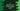# C program to print the size of the longest word in a string## C program to print the size of the longest word in a string:

In this post, we will write one program that will print the size of the longest word in a string in C. The program will take one string as input from the user and print out the size of the longest word.

For example, if the string is hello Universe, it will print 8 as this is the length of the longest word Universe.

With this program, you will learn how to read a string in C, how to iterate through the characters of a string and how to find the length of the longest word.

### Algorithm to use:

We will use the below algorithm in this program:

• Read the string from the user.
• Iterate through the characters of the string one by one from start to end.
• Initialize one variable to hold the length of the longest word and to hold the length of the current word. Initialize both to 0.
• For each character, check if it is a blank character or not.

• If it is not a blank character, increment the value of the variable that holds the length of the current word by 1. Because it is a word that we are iterating its character.
• If it is a blank character, that means it is the end of a word. Compare the current word length with the maximum word length variable. If it is more than the maximum word length value, assign its value to maximum word length. Also assign 0 to the current word length variable and more to next iteration.
• The loop will end once it reaches the end of the string. We have to do one more comparison of current and maximum word length for the last word of the string.
• Once the loop ends, print the value of the maximum word length calculated.

### C program:

Let’s take a look at the C program first:

``````#include <stdio.h>

int main()
{
int currentWordLength = 0;
int maxWordLength = 0;
char input;

printf("Enter a string:\n");
scanf("%[^\n]", input);

for (int i = 0; input[i] != '\0'; i++)
{
if (input[i] != ' ')
{
currentWordLength++;
continue;
}

if (currentWordLength > maxWordLength)
{
maxWordLength = currentWordLength;
}

currentWordLength = 0;
}

if (currentWordLength > maxWordLength)
{
maxWordLength = currentWordLength;
}

printf("Length of the longest word: %d", maxWordLength);

return 0;
}``````

### Explanation:

In this program,

• currentWordLength variable is to hold the current word length.
• maxWordLength variable is to hold the maximum word length.
• input is a character array to hold a user string. We are using scanf to read the string entered by the user and it is stored in input.
• The for loop is to iterate through the characters of the string one by one. It starts from the character at 0th index, i.e. at the first character and it ends the iteration at the end character i.e. at \0.

• The first if statement inside the loop checks if the current character is a whitespace character or not. If it is not a whitespace character, it increases the value of the currentWordLength variable by 1. The continue statement skips the below part and starts the next iteration.
• The second if statement checks if the value of currentWordLength is greater than maxWordLength or not. This statement is reached only if the loop reached the end of a word, i.e. only if the current character is not blank.
• After the loop, we are using one more if condition to check if the value of currentWordLength is greater than maxWordLength or not. This line checks the last word of the string.
• The last printf statement prints the value of maxWordLength, i.e. the longest word length.

### Sample output:

If you run this program, it will print output as like below:

``````Enter a string:
hello world
Length of the longest word: 5

Enter a string:
hello universe
Length of the longest word: 8

Enter a string:
a b c de fgh ijkl m
Length of the longest word: 4``````